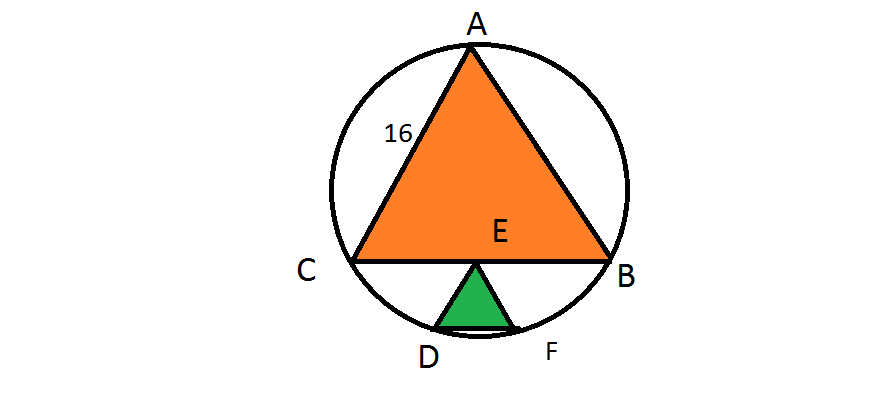Two similar equilateral triangles in a circle

Geometry Level 4Two equilateral triangles reside in a circle, one on top of the other as shown in the figure above.

If $E$ is the midpoint of the side $BC$ of the larger orange triangle which has a side length of $16$ and side $DE$ of the smaller triangle is parallel to $AC$, find the length of $DE$ correct to 2 decimal places.

×